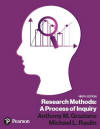﻿ Pearson Correlation in SPSS for WindowsGraziano & Raulin
Research Methods (9th edition)

## Pearson Product-Moment Correlation

### Computing a Correlation

To compute the Pearson product-moment correlation between age and income, we select the Analyze menu, the Correlate submenu, and the Bivariate option (which literally means two variables). These selections will give us this screen

We select the variables age and income and move them to the right box. The default options in this procedure are to compute a Pearson product-moment correlation and a two-tail p-value. Since these are the options we want, we only need to click on OK to run the correlations. The resulting output is shown in this screen

Note that SPSS for Windows actually gives us four correlations. The program is set up to compute an intercorrelation matrix (i.e., the correlation of each variable with every other variable that was selected) for as many variables as you select. If you select 10 variables, there would be a 10 by 10 matrix of intercorrelations. The diagonal (from the upper left to the lower right) of that matrix will list the correlation of each variable with itself, which will always be equal to 1.00 (a perfect correlation). The correlational matrix above and below this diagonal will be mirror images of one another,  because the correlation of variable X with variable Y is the same as the correlation of variable Y with variable X

In our simplified example of only two variables, the correlation between age and income is .883 and the probability of getting that correlation or a larger correlation by chance if the population correlation were actually zero (the p-value) is listed as .000. Actually, no correlation will give you a p-value of zero; but since only three digits are printed, a value listed as .000 actually means that the probability is less than .001. If we assume the traditional decision criteria (termed alpha level) of .05, we would conclude that these two variables are significantly correlated with one another, because our p-value is less than the alpha level of .05 (see Chapter 5 for a more detailed explanation of this terminology).

### Producing a Scatter Plot

Remember that a Pearson product-moment correlation is an index of the degree of linear relationship between two variables. That is, the correlation gives an indication of how closely the points in a scatter plot cluster around a straight line. But the relationship between two variables is not always linear. For example, if we correlated a measure of general health with weight, we would likely find that people who are either excessively heavy or light would have generally poor health and that people in the middle range of weight would likely be the healthiest.

To see what the shape of a relationship is like, you would prepare a scatter plot. SPSS for Windows will prepare a scatter plot with just a few mouse clicks. Click on the Graphs menu, Interactive submenu, and select the Scatterplot option. These actions will produce this screen. To prepare a scatter plot of age by income, move the income variable to X axis and then move the age variable to the Y axis of the graph shown. Clicking on the OK button will produce the scatter plot. As you can see, the relationship between age and income does appear to be linear in our data set.

### Animations

We have prepared a series of animations that will walk you through the procedures discussed on this page. To run an animation, simply click on the title of the animation in the table below.

Note that we do not recommend that you try to run the animations if you have a slow connection, such as a dial-up connection. You will find that the animations take forever to load with a slow connection.

 Computing a Product-Moment Correlation Producing a Scatter Plot